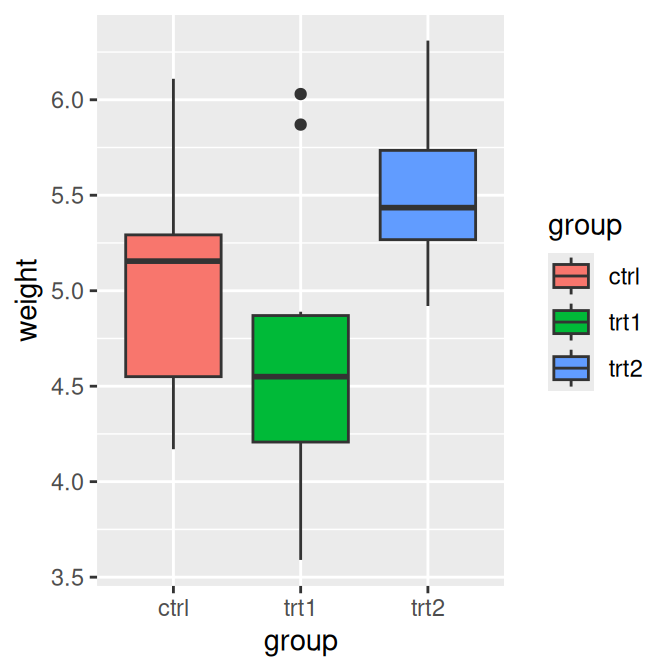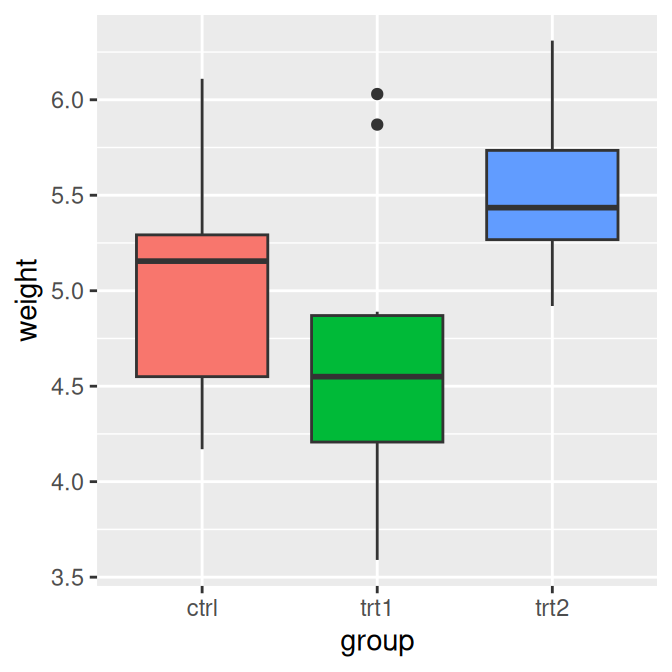## 10.1 Removing the Legend

### 10.1.1 Problem

You want to remove the legend from a graph.

### 10.1.2 Solution

Use `guides()`, and specify the scale that should have its legend removed (Figure 10.1):

``````# Create the base plot (with legend)
pg_plot <- ggplot(PlantGrowth, aes(x = group, y = weight, fill = group)) +
geom_boxplot()

pg_plot

# Remove the legend for fill
pg_plot +
guides(fill = FALSE)
#> Warning: The `<scale>` argument of `guides()` cannot be `FALSE`. Use "none" instead
#> as of ggplot2 3.3.4.
#> This warning is displayed once every 8 hours.
#> Call `lifecycle::last_lifecycle_warnings()` to see where this warning was
#> generated.``````Figure 10.1: Default appearance (left); With legend removed (right)

### 10.1.3 Discussion

Another way to remove a legend is to set `guide = FALSE` in the scale. This will result in the exact same output as the preceding code:

``````# Remove the legend for fill
pg_plot +
scale_fill_discrete(guide = FALSE)``````

Yet another way to remove the legend is to use the theming system. If you have more than one aesthetic mapping with a legend (`color` and `shape`, for example), this will remove legends for all of them:

``````pg_plot +
theme(legend.position = "none")``````

Sometimes a legend is redundant, or it is supplied in another graph that will be displayed with the current one. In these cases, it can be useful to remove the legend from a graph.

In the example used here, the colors provide the same information that is on the x-axis, so the legend is unnecessary. Notice that with the legend removed, the area used for graphing the data is larger. If you want to achieve the same proportions in the graphing area, you will need to adjust the overall dimensions of the graph.

When a variable is mapped to `fill`, the default scale used is `scale_fill_discrete()` (equivalent to `scale_fill_hue()`), which maps the factor levels to colors that are equally spaced around the color wheel. There are other scales for `fill`, such as `scale_fill_manual()`. If you use scales for other aesthetics, such as `colour` (for lines and points) or `shape` (for points), you must use the appropriate scale. Commonly used scales include:

• `scale_fill_discrete()`
• `scale_fill_hue()`
• `scale_fill_manual()`
• `scale_fill_grey()`
• `scale_fill_brewer()`
• `scale_colour_discrete()`
• `scale_colour_hue()`
• `scale_colour_manual()`
• `scale_colour_grey()`
• `scale_colour_brewer()`
• `scale_shape_manual()`
• `scale_linetype()`